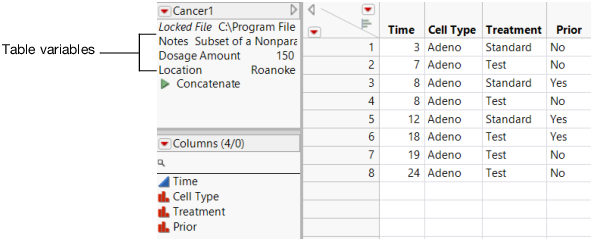Using JMP > Enter and Edit Your Data > Edit Data Tables > Use Data Table Variables
Publication date: 11/29/2021

## Use Data Table Variables

A data table variable can contain textual information (for example, source information for the data), or a value that can be used by column formulas or JSL scripts. Data table variable names appear in the table panel at the left of the data grid.

Figure 4.61 Table Variables in the Table Panel### Uses for Table Variables

Use table variables in the following situations:

To document tables

In formulas

In JSL scripts

#### Use Table Variables to Document Tables

Table variables are used primarily to document tables. Many sample data tables installed with JMP contain a table variable named Notes. This variable provides details about the data (for example, the source of the data). The example in Figure 4.61 shows a data table that contains Notes as one of its table variables. JMP also automatically creates table variables when you create a design table using the Design of Experiments commands in JMP. The design table has a table variable named Design with the name of the design type as its value.

#### Reference Table Variables in Formulas

Table variables can also be incorporated in formulas that you build using the Formula Editor. These formulas calculate values for a column by referring to a table variable. For more information about constructing a formula that uses table variables, see Refer to Data Table Values in Formulas.

#### Use Data Table Variables in JSL Scripts

You can also incorporate table variables into JSL scripts. See Data Table Variables in the Scripting Guide.

### Data Table Variable Actions

To add new data table variables

1. In the Table panel, click the red triangle to the left of the data table name.

2. Select New Table Variable.

3. Give the variable a name and value in the boxes labeled Name and Value.

4. Click OK.

The table variable appears in the Table panel.

To view or edit table variables

1. Double-click the content of an existing table variable.

2. Edit the content.

To edit a table variable name

1. Double-click the table variable name.

2. Edit the name.

To delete table variables

Select one or more table variables and press Delete, or right-click the selected variables and select Delete.